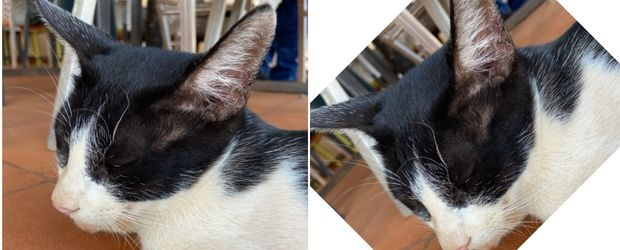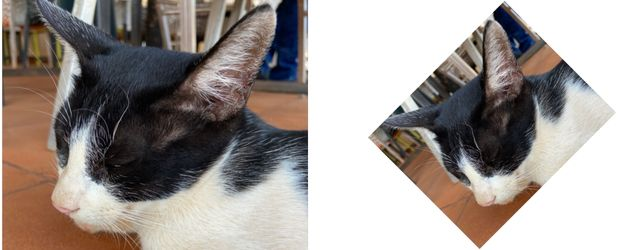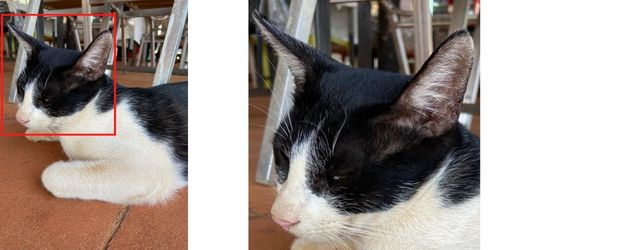# Python Imaging Library PIL

``pip install Pillow``

Python Imaging Library: Pillow basics to create or read images to resize, rotate, generate thumbnail

## Creating a new Image

``img = Image.new(mode, size, color)``
mode: RGB or RGBA
size: Tuple (width,height) of the image.
color : yellow , green or other supported colours OR Hex value
``````from PIL import Image
path = "F:\\testing\\images\\test3.png"  # new image to create
img = Image.new("RGB", (400, 200), color="#F1F1CC")
img.save(path)``````

## Saving Image

In above code we used the file name and path to save the image.
``Image.save(path, format=None, **params)``

## Opening Image

``Image.open(path, mode='r', formats=None)``
path: Path of the image to open
mode : 'r' for read mode
formats : A list of tuple of formats.
``````from PIL import Image
path = "F:\\testing\\images\\cat.png"  # existing image to open
img = Image.open(path, mode="r")
img.show()``````

## Getting image details

``````path = "F:\\testing\\images\\cat.png"  # existing image to open
img = Image.open(path)
print(img.format)  # Image format
print(img.mode)  # pixel mode RGBA
print(img.size)  # ( 620,500)
print(img.height)  # 500
print(img.width)  # 620
print(img.info)  # all details about the image.``````

## Rotate Image``````from PIL import Image
path = "F:\\testing\\images\\cat.png"  # existing image to open
path2 = "F:\\testing\\images\\cat2.png"  # Path to save
img = Image.open(path)
img2 = img.rotate(45)  # rotate by 45 degree
img2.show()
img2.save(path2) # save rotated image ``````
Retaining the size after rotate.
``img2 = img.rotate(45, expand=True)  # rotate by 45 degree``## Resizing Images

``````Image.resize(size, resample=None, box=None, reducing_gap=None)[source]
``````
size: Tuple (width, height), required size.
resample : optional resampling filter
box : optional, for the region to be scaled. (0,0,width,height)
reducing_gap : Apply optimization by resizing the image in two steps.
``````from PIL import Image

path = "F:\\testing\\images\\jump.jpeg"  # Path to Image
path2 = "F:\\testing\\images\\jump_resize.jpeg"  # resized
img = Image.open(path, mode="r")  # create image object
img.show()  # display
img_new = img.resize((200, 200))  # resize the image
print(img.size)  # original image size ( width , height)
print(img_new.size)  # resized image size ( width, height)
img_new.show()  # display resized image
img_new.save(path2)  # save resized image``````

## Thumbnail

To maintain aspect ratios we have to use thumbnail(). We can also give maximum width and maximum height (as tuple) of the thumbnail image.
``````from PIL import Image

path = "F:\\testing\\images\\jump.jpeg"  # Path to Image
path2 = "F:\\testing\\images\\jump_thumbnail.jpeg"  # resized
img = Image.open(path, mode="r")  # create image object
img.show()  # display
print(img.size) # main image size ( width, height)
img.thumbnail((200, 200))  # max width, max height
img.save(path2)  # save thumbnail  image
print(img.size)  # thumbnail size ( width, height)
img.show()  # display resized image``````
Above code will print two outputs as sizes, note how the aspect ratio is maintained while creating Thumbnail image.
``````(1024, 768)
(200, 150)``````

## Image CropTo crop an image we have to use crop()
``Image.crop(box=None)``
box : the rectangle or tuple( left,upper, right, lower )
``````from PIL import Image

path = "F:\\testing\\images\\cat-crop.jpeg"  # Path to Image
path2 = "F:\\testing\\images\\cat-crop2.jpeg"  # new image
img = Image.open(path, mode="r")  # create image object
img.show()  # display
print(img.size)  # main image size ( width, height)
box = (20, 65, 470, 550)  # rectangle for crop
img_crop = img.crop(box)  # Cropped image object
img_crop.save(path2)  # save cropped image
print(img_crop.size)  # cropped image size ( width, height)
img_crop.show()  # display cropped  image``````
Check the size of images, compare with the rectangle ( box ) input given to the crop().
``````(768, 1024)
(450, 485)``````

Subscribe to our YouTube Channel here

## Subscribe

* indicates required
Subscribe to plus2netplus2net.com